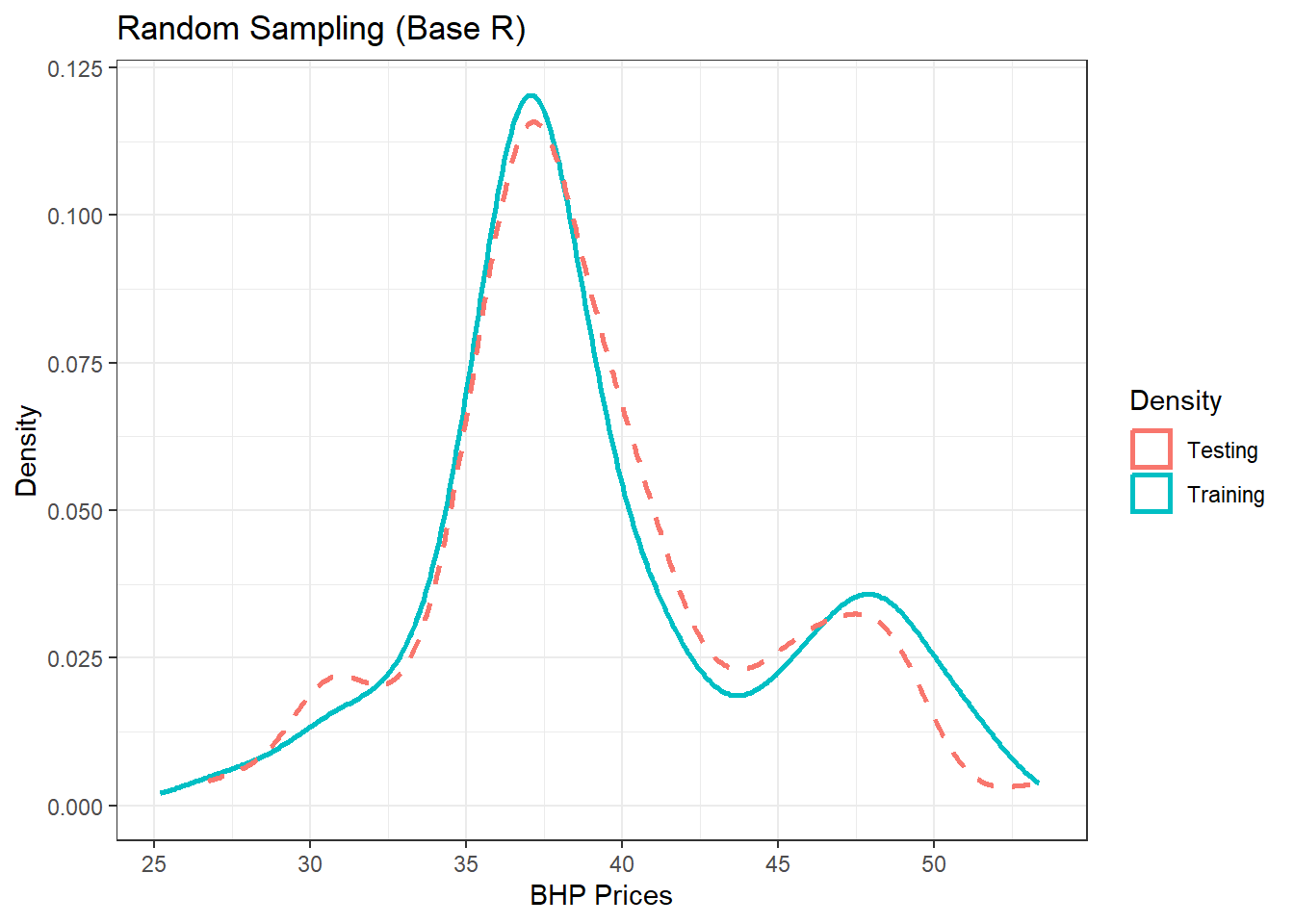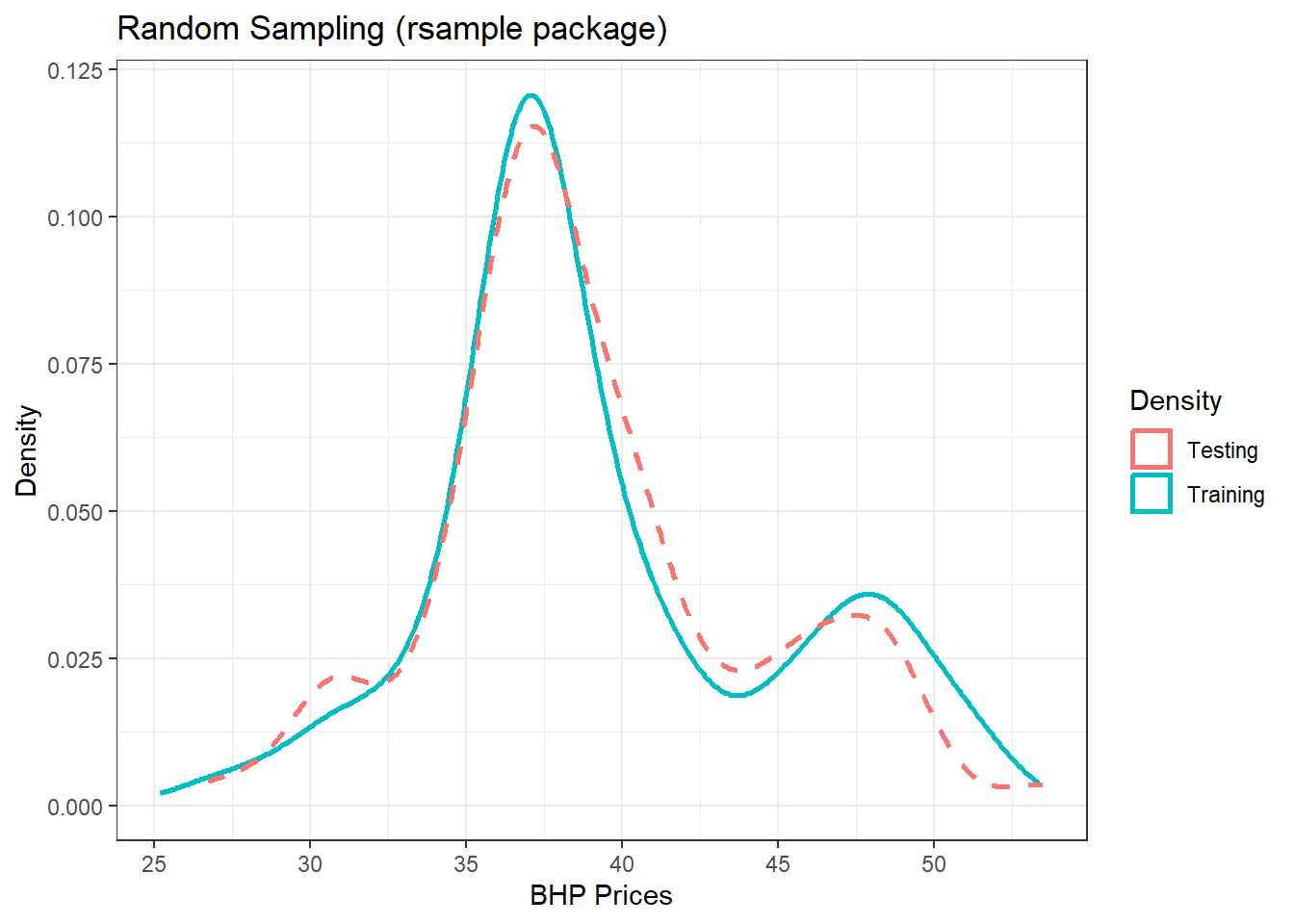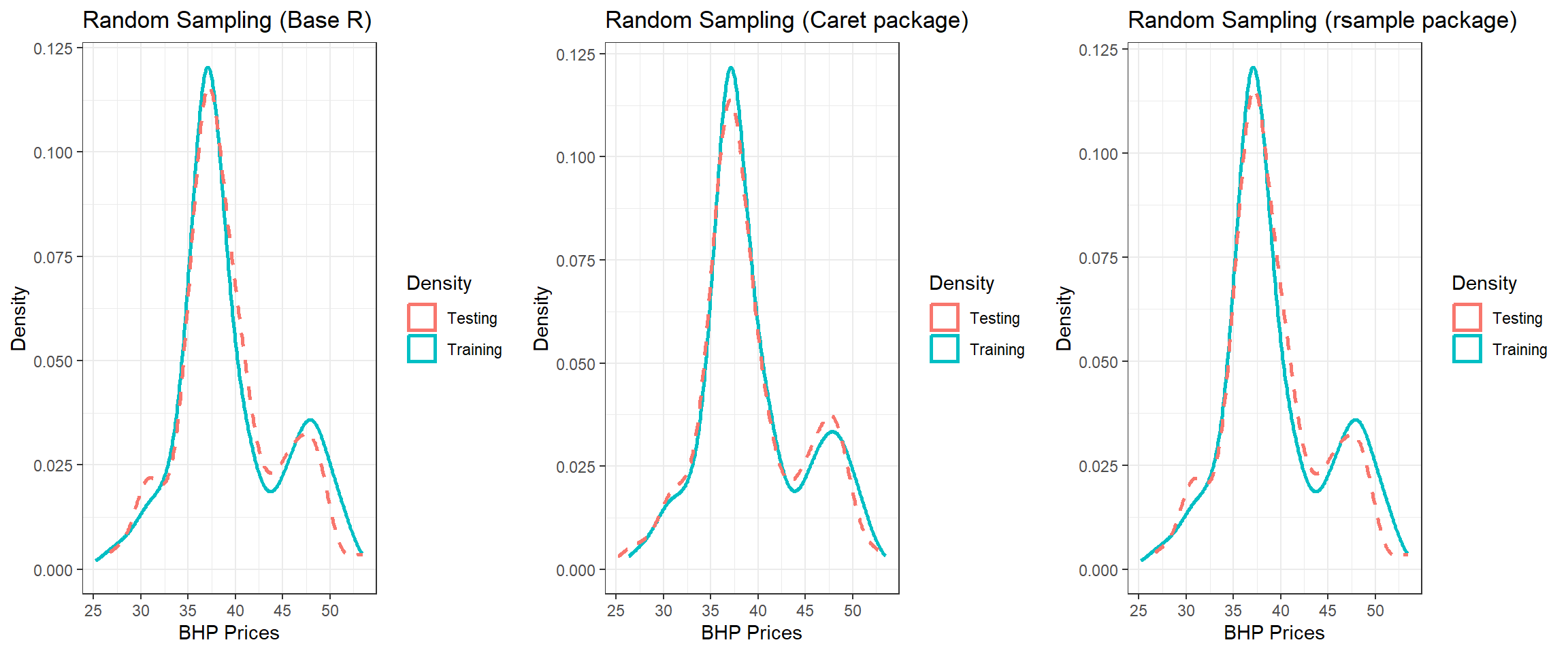## 13.3 Random Sampling

This section explores some ways to conduct random sampling in R. Simple Random sampling does not control for any data attributes.

### 13.3.1 Base R

The following code uses the BHP close prices to perform a simple random sample using base R sample function.

# import data and select the closing prices
library(xts)  #required as the data was saved as an xts object
d_bhp = d_bhp$BHP.AX.Close #select close prices d_bhp = data.frame(Date = as.Date(index(d_bhp)), Price = coredata(d_bhp)) #convert to data frame (for convenience not necessaily required) head(d_bhp)  Date BHP.AX.Close 1 2019-01-02 33.68 2 2019-01-03 33.68 3 2019-01-04 33.38 4 2019-01-07 34.39 5 2019-01-08 34.43 6 2019-01-09 34.30 # use base R function set.seed(999) #seed is set for reproducibility as the random number generator picks a different seed each time unless specified idx1 = sample(1:nrow(d_bhp), round(nrow(d_bhp) * 0.7)) #70% # training set train1 = d_bhp[idx1, ] # testing set (remaining data) test1 = d_bhp[-idx1, ] Note: Sampling is a random process and random number generator produces different results on each execution. Setting a seed in the code keeps it consistent allows for reproducibility. • Visualise the distribution of training and testing set library(ggplot2) p1 = ggplot(train1, aes(x = BHP.AX.Close)) + geom_density(trim = TRUE, aes(color = "Training"), size = 1) + geom_density(data = test1, aes(x = BHP.AX.Close, color = "Testing"), trim = TRUE, size = 1, linetype = 2) (p1 = p1 + theme_bw() + labs(color = "Density", title = "Random Sampling (Base R)", x = "BHP Prices", y = "Density"))Figure 13.1: Training/Testing using Base R ### 13.3.2 Using the caret package • We can use the caret package to create the training and testing samples set.seed(999) library(caret) idx2 = createDataPartition(d_bhp$BHP.AX.Close, p = 0.7, list = FALSE)
train2 = d_bhp[idx2, ]
test2 = d_bhp[-idx2, ]

# plot
p2 = ggplot(train2, aes(x = BHP.AX.Close)) + geom_density(trim = TRUE,
aes(color = "Training"), size = 1) + geom_density(data = test2, aes(x = BHP.AX.Close,
color = "Testing"), trim = TRUE, size = 1, linetype = 2)
(p2 = p2 + theme_bw() + labs(color = "Density", title = "Random Sampling (Caret package)",
x = "BHP Prices", y = "Density"))Figure 13.2: Training/Testing using caret

### 13.3.3 Using the rsample package

• Provides an easy to use method for sampling which is slightly different but can be more convenient due to the function names
set.seed(999)
library(rsample)
idx3 = initial_split(d_bhp, prop = 0.7)  #creates an object to further use for training and testing

train3 = training(idx3)
test3 = testing(idx3)

# plot

p3 = ggplot(train3, aes(x = BHP.AX.Close)) + geom_density(trim = TRUE,
aes(color = "Training"), size = 1) + geom_density(data = test3, aes(x = BHP.AX.Close,
color = "Testing"), trim = TRUE, size = 1, linetype = 2)

(p3 = p3 + theme_bw() + labs(color = "Density", title = "Random Sampling (rsample package)",
x = "BHP Prices", y = "Density"))Figure 13.3: Training/Testing using rsample

Combine all three plots

• Notice some differences between the three due to the method used.
library(gridExtra)
grid.arrange(p1, p2, p3, nrow = 1)Figure 13.4: Splitting using three methods